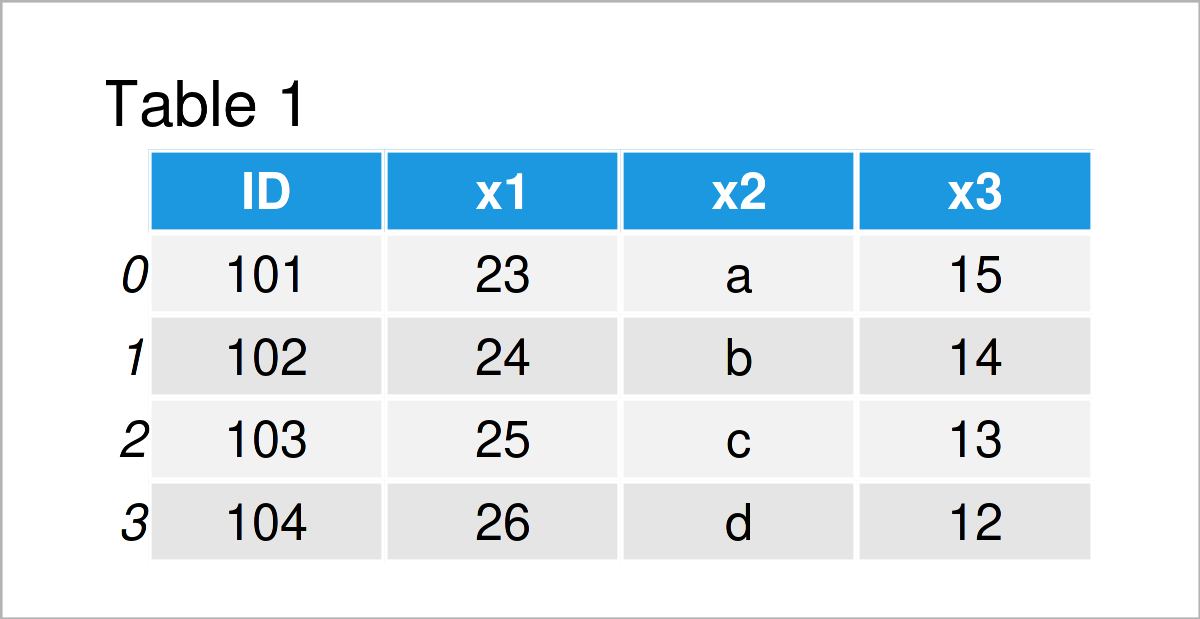# Merge pandas DataFrames in CSV Files in Python (Example)

This tutorial demonstrates how to join pandas DataFrames in different CSV files into a single file in Python.

The article contains these content blocks:

With that, let’s do this.

## Exemplifying Data & Add-On Libraries

First, we need to import the pandas library:

`import pandas as pd                                 # Load pandas library`

We also need to construct two example DataFrames:

```data1 = pd.DataFrame({'ID':range(101, 105),         # Create first pandas DataFrame
'x1':range(23, 27),
'x2':['a', 'b', 'c', 'd'],
'x3':range(15, 11, - 1)})
print(data1)                                        # Print first pandas DataFrame````data1.to_csv('data1.csv', index = False)            # Export first pandas DataFrame`
```data2 = pd.DataFrame({'ID':range(103, 107),         # Create second pandas DataFrame
'y1':range(27, 23, - 1),
'y2':['x', 'y', 'x', 'y']})
print(data2)                                        # Print second pandas DataFrame````data2.to_csv('data2.csv', index = False)            # Export second pandas DataFrame`

After executing the previous Python programming syntax the two pandas DataFrames shown in Tables 1 and 2 have been created and exported as CSV files.

Next, I’ll show how to merge these two data sets into one single CSV file

## Example: Read, Merge & Export pandas DataFrames in CSV Files

The following syntax explains how to import, combine, and export two pandas DataFrames from two CSV files to a single file.

In the first step of this example, we have to load the two data sets using the read_csv function:

```data1_import = pd.read_csv('data1.csv')             # Read first CSV file

Next, we can merge our two DataFrames as shown below. Note that we are using a full outer join in this specific example. However, we could apply any other kind of join that we want.

```data_merge = pd.merge(data1_import,                 # Full outer join
data2_import,
on = "ID",
how = "outer")
print(data_merge)                                   # Print merged DataFrame```In Table 3 it is shown that we have created a new pandas DataFrame containing the values of both our input data sets.

In the final step, we can write the merged pandas DataFrame to a new CSV file using the to_csv function:

`data_merge.to_csv('data_merge.csv', index = False)  # Export merged pandas DataFrame`

After executing the previous Python syntax, a new CSV file will appear in your current working directory.

Please note: We have merged only two pandas DataFrames in this tutorial. However, we could also use this syntax to combine multiple DataFrames.

## Video, Further Resources & Summary

Do you need further info on the Python programming syntax of this tutorial? Then you may watch the following video on my YouTube channel. In the video, I’m demonstrating the Python codes of this page in a live session:

In addition, you may want to read the related tutorials on this website. Some articles are listed below:

In summary: You have learned in this tutorial how to merge pandas DataFrames in multiple CSV files in the Python programming language. If you have any further questions, tell me about it in the comments.

Subscribe to the Statistics Globe Newsletter